Transcripted Summary

In this chapter, we will see assertion styles of Chai's interfaces.

So, we have got Chai already, and now we can start using that.

I'm using WebStorm IDE and opening the project on it. You can use any JavaScript editor you wish to use.

I have a test folder in this project. Inside that, I am creating a JavaScript file called `chai.js`.

To get the Chai interfaces we need to import Chai to this file, which can be done by creating a variable:

``````const chai = require('chai');`
``````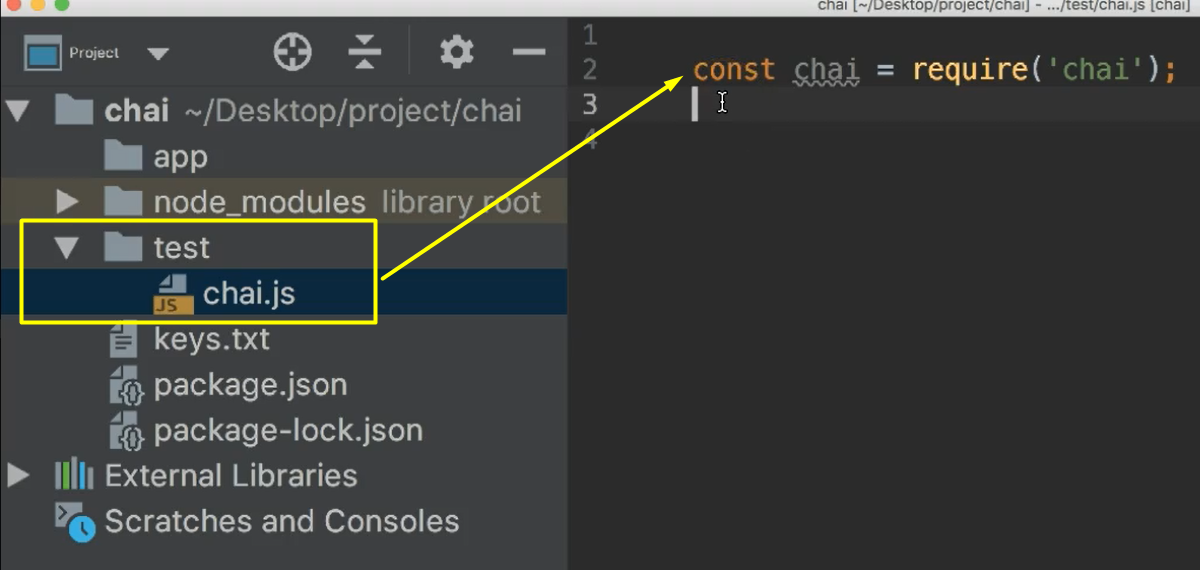### # Assertion Style - Expect

Now the first interface that we are going to look at is `expect`. As mentioned earlier, `expect` provides an expressive language and a readable style by chaining certain keywords. The keywords are shown below.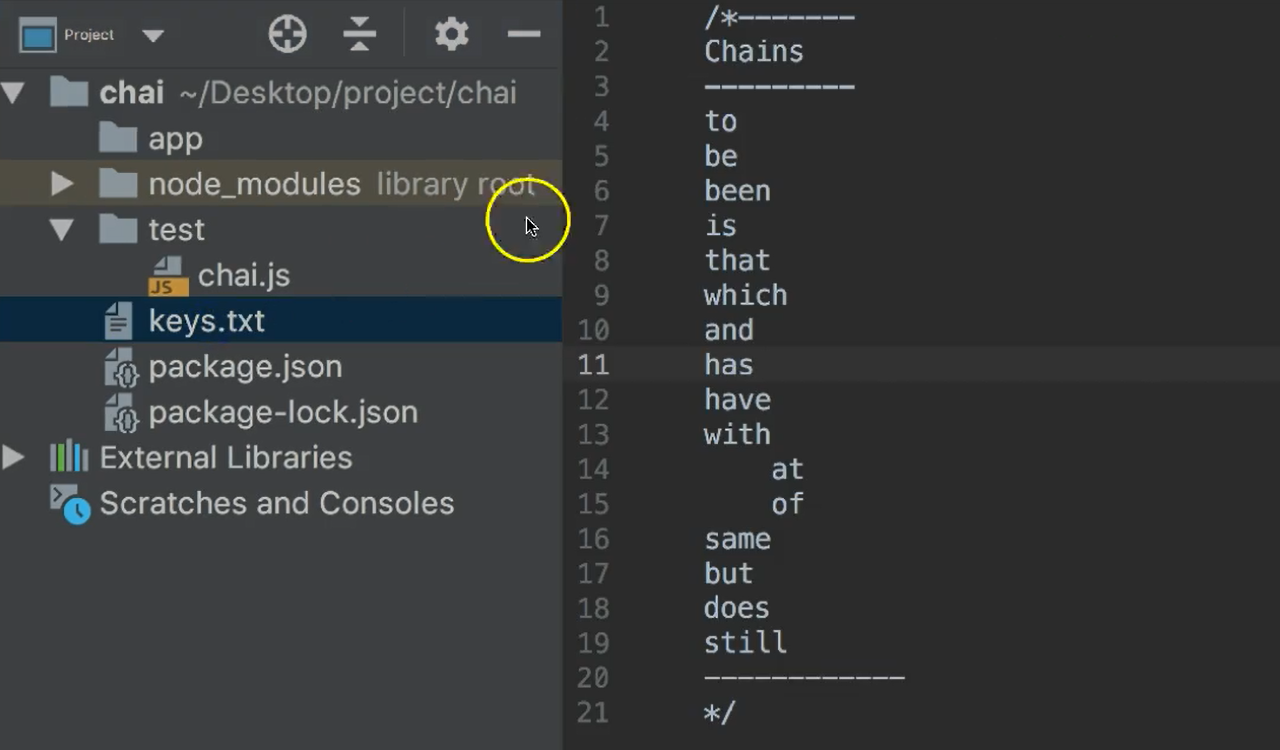Let's see some examples now.

Create another variable as follows:

``````//expect
const expect = chai.expect;
``````

Please note that we are getting `expect` from Chai, which is defined in the previous step.

The `expect` interface provides lots of API's to do assertion. We will go through some of them.

Let's see the first example — consider there are 2 numbers, `a` and `b`, having values 1 and 2.

We will see how we use `expect` to validate that `a` and `b` are equal.

The syntax of the keyword `expect` takes an actual value as an argument, then dot, and we can use any of the styles that are listed.

I will use `to.be.equals` then that takes another argument of the expected value that wants, `a` to be equal to `b`.

``````//expect API example – equals with failed test
let a=1, b=2;
expect(a).to.be.equals(b);
``````

This gives a nice readable style to that expression.

On the terminal, let us execute the test now.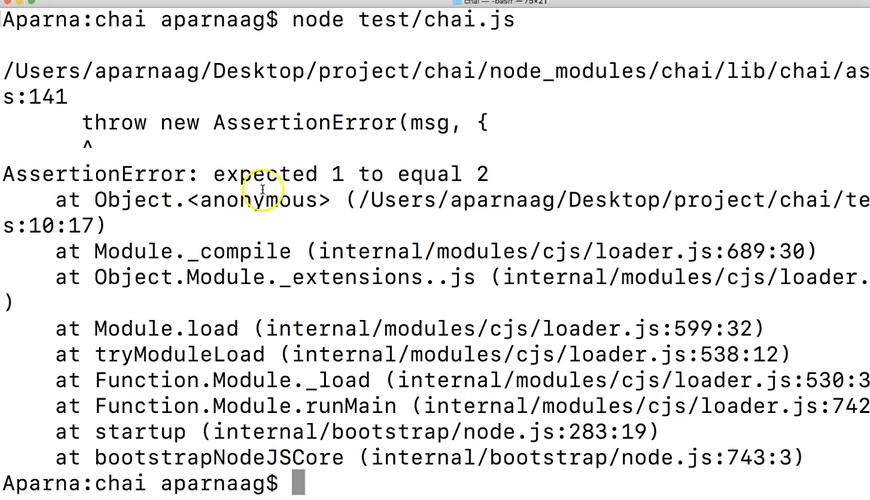We will get error as `a` and `b` are not equal.

Coming back to the IDE, we can provide a meaningful message, by passing an argument along with the expected string. Something like, "a and b are not equal".

So, the revised expression will look like:

``````//expect API example – equals with failed test error message
let a=1, b=2;
expect(a).to.be.equals(b, "a and b are not equal");
``````

Moving to the terminal, lets rerun this script again.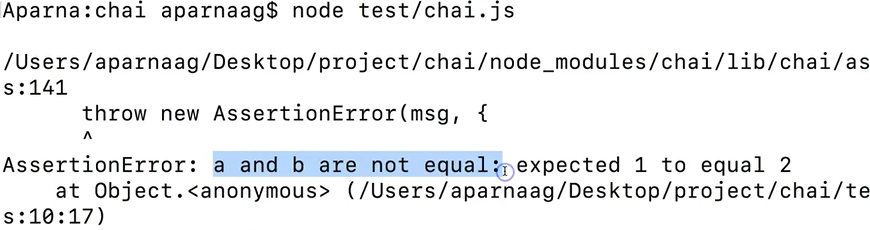See now it's printing the message that gives more meaningful message to the assertion error.

Now, on the IDE, I will change the value of `b` to 1, and rerun the script on terminal.

``````//expect API example – equal passing test
let a=1, b=1;
expect(a).to.be.equals(b, "a and b are not equal");
``````

Here, we see a solution has passed, and no error was drawn.

Similarly, we can validate whether a specific input is an object, or a String, or a number, or a Boolean, etc.

We will see a couple of them.

Now, I'm creating a function called `myOBJ` which returns a String and a number, with `a` being 100, and `b` being “hello”.

For this function, we will create 2 objects, `x` and `y`. Now let’s validate whether `x` is an object using `expect`.

``````//expect - object/string/boolean
function myObj(){
return{
a: 100,
b: 'Hello'
}
}

x = new myObj(), y = new myObj();
expect(x).to.be.an('object');
``````

Let’s execute this script on the terminal. See? It's passing.

Now let us see if we can check the equality of `x` and `y`.

We know the syntax already. I'm just copying the above command and modifying it.

``````//expect - object/string/boolean – with equality check
function myObj(){
return{
a: 100,
b: 'Hello'
}
}

x = new myObj(), y= new myObj();
expect(x).to.be.an('object');
expect(x).to.be.equals(y, 'x and y are not equal');
``````

Run it. See, assertion is failing with the error.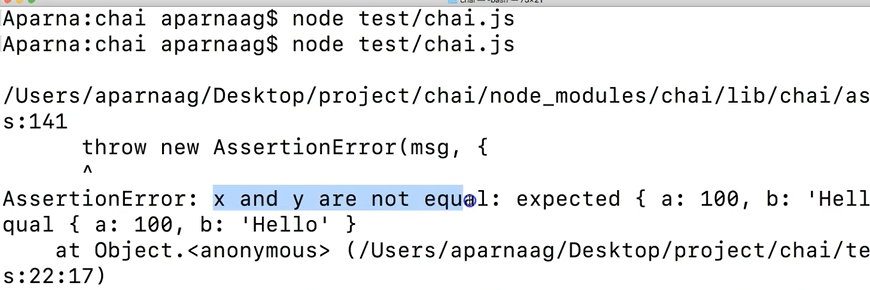This means that when we use `equal` or `equals` it is not looking at the content of the object.

To compare object contents, we should use something called `deep.equal`, or `deep.equals`.

So, copying the above one and modifying into — `expect(x).to.be.deep.equals` — and we can also pass a message, "x and y are not equal".

``````//expect - deep.equal or deep.equals
expect(x).to.be.deep.equals(y, 'x and y are not equal');
``````

Run it. Yes. That passes.

Now, let us look at how to chain different expressions.

The beauty of `expect` is that it provides an option to chain multiple `expect` expressions together.

For example, if we need to check whether `x` is an object also, whether `x` and `y`. are equal. How can we do it?

We have certain keywords for that as well — `and`, `but`, `with`, et cetera.

We will revise our expression now.

Copy the one which is verifying whether `x` is an object and add: `and.to.be.deep.equals(y)`.

``````//expect - chaining expressions
expect(x).to.be.an('object').and.to.be.deep.equals(y);
``````

It is always a good practice to use `a` or `an` to check target type before performing assertion on it. This will avoid unexpected behavior or errors that may occur when performing the assertions on the target type. So, chaining helps in such scenarios.

Let us now look at another example, where we check the target is an array, and which has a specific value in it.

Create an array called "numbers", which has numbers "1, 2, 3, and 0". Then, expect “numbers” to be an array. Let’s look for number 3.

``````//arrays
let numbers = [1,2,3,0];
expect(numbers).to.be.an('array').that.includes(3);
``````

Execute the test. It's running without any error.

But, if I change the number 3 to 4 — `.that.includes(4)` —, where 4 is not in the array, we will get an error.

Run it. There we go.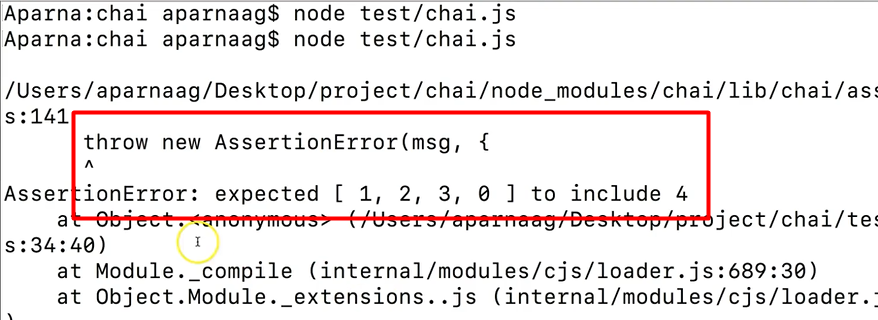It says, "Assertion:Error: expected [1,2,3,0] to include 4”.

Alright, so we covered few of the `expect` styles. There are a lot more styles that can be referred in Chai's official website page. I'll provide a link in the resources here, and at the end of the course.

### # Assertion Style - Should

Now, let us look at the next BDD style Chai interface, which is `should`. Though `expect` and `should` are BDD styles, and provide expressive readable styles, they are different.

The main difference is that `should` extends each object with a `should` property to start the chain.

Let us look at the examples.

To import `should` we use the following command:

``````//should()
const should = chai.should();
``````

So, our first example for simple comparison of 2 numbers can be rewritten using `should` as follows.

``````//should - simple comparison
a.should.be.equals(b);
``````

Similarly, object comparison will be like this — object `equal` will throw an assertion error, because `equal` will not compare content of the object — so we will have to use the `deep.equals` with `should`.

So, the expression for object comparison becomes:

``````//should – object comparison with deep.equal or deep.equals
x.should.be.deep.equals(y, 'x and y are not equal');
``````

Now, we will see how to chain should expressions.

`x` is an object and `x` and `y` are equal. We can write like this.

``````//should - chaining expressions
(x).should.be.an('object').and.to.be.deep.equals(y);
``````

And if I change “object” to “number” — `.should.be.an('number')` — that throws assertion error.

So, lets execute the `should` expressions and see the results.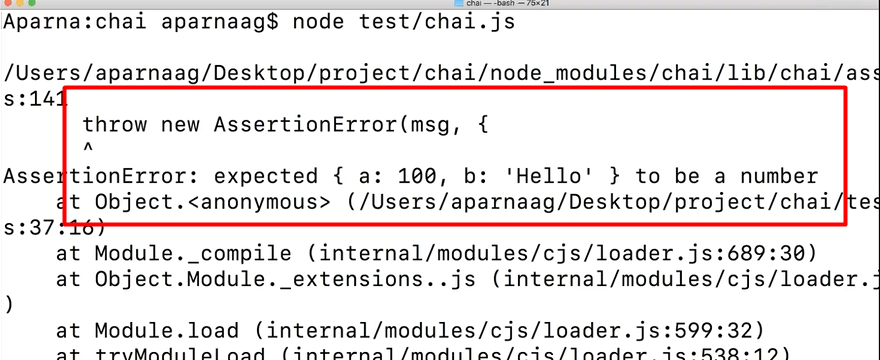See the assertion is failing for the number check. Okay, here, I am changing the “number” back to “object”.

Now we can look at array comparison with `should`.

So, “numbers” as an array that dot includes number 3.

``````//should - arrays
(numbers).should.be.an('array').that.includes(3);
assert.isArray(numbers, 'numbers is not an array');
``````

With this, we covered a few examples with `should` as well. Let us execute all of them.

All good. No assertion errors.

Before looking into differences between `expect` and `should`, let us cover `assert`.

### # Assertion Style - Assert

As mentioned before, `assert` is something similar to Node JS default `assert` method and has a classic TDD style.

Let us define assert as follows.

``````//Assert - TDD style
const assert = chai.assert;
``````

One thing to be noted is that using `assert` we cannot chain expressions as we do with `expect` and `should`.

Now we will go through examples.

We will rewrite few examples that were written with `expect` and `should`.

Comparison of two numbers will be:

``````//assert – simple comparison
assert.equal(a,b, "a and b are not equal");
``````

Similarly, object comparison can be rewritten using `deepEqual`.

``````//assert – object comparision
assert.deepEqual(x,y, "x and y are not equal");
``````

The array example will be:

``````//assert – arrays
(numbers).should.be.an('array').that.includes(3);
assert.isArray(numbers, 'numbers is not an array');
``````

Now, go to Terminal, we will run all of these examples and see if there are any errors.

So, this is clean and no error.

## Resources

Quiz

The quiz for this chapter can be found in section 3.2